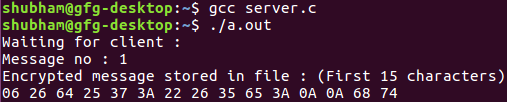Open in App
Not now

# Message encryption and decryption using UDP server

• Difficulty Level : Expert
• Last Updated : 26 Jul, 2021

The process of message encryption and decryption during client-server communication using UDP server is as follows:

• The client requests the server with a file name.
• The corresponding file is opened by the server and sends the file using datagram socket.
• The sender sends the encrypted text (Xoring) with a fixed length key.
• The receiver decrypts the file using the same key (private key).

Prerequisite : Socket Programming

Examples:

```Input :  file.txt
Output : ClientSide : Received from server:
00 2C 28 28 2A 6E 3B 24 37 64 65 79 0A 00 00
Decrypted message: Hello People
ServerSide : Encrypted message stored in file:
00 2C 28 28 2A 6E 3B 24 37 64 65 79 0A 00  ```

Client Side Program:

## C

 `// Client side code``#include ``#include ``#include ``#include ` `// Driver code``int` `main()``{``    ``int` `clientSocket, portNum, nBytes;``    ``char` `file_buffer, path, buffer;` `    ``// This key array stores the hidden key``    ``char` `const` `key = ``"HIDDENKEY"``;``    ``struct` `sockaddr_in serverAddr;``    ``socklen_t addr_size;``    ``int` `i;``    ``clientSocket = socket(PF_INET, SOCK_DGRAM, 0);` `    ``serverAddr.sin_family = AF_INET;``    ``serverAddr.sin_port = htons(5004);``    ``serverAddr.sin_addr.s_addr = inet_addr(``"127.0.0.1"``);``    ``memset``(serverAddr.sin_zero, ``'\0'``, ``sizeof` `serverAddr.sin_zero);` `    ``addr_size = ``sizeof` `serverAddr;` `    ``while` `(1) {``        ``printf``(``"Specify file name: \n"``);``        ``gets``(path);` `        ``// printf("%s\n", path);``        ``FILE``* fp;``        ``fp = ``fopen``(path, ``"r"``);``        ``if` `(fp == NULL) {``            ``printf``(``"file does not exist\n"``);``        ``}` `        ``fseek``(fp, 0, SEEK_END);``        ``size_t` `file_size = ``ftell``(fp);``        ``fseek``(fp, 0, SEEK_SET);` `        ``if` `(``fread``(file_buffer, file_size, 1, fp) <= 0) {``            ``printf``(``"unable to copy file into buffer\n"``);``            ``exit``(1);``        ``}` `        ``if` `(sendto(clientSocket, file_buffer, 3000, 0, (``struct` `sockaddr*)&serverAddr,``                                                                    ``addr_size) < 0) {``            ``printf``(``"error in sending the file\n"``);``            ``exit``(1);``        ``}` `        ``bzero(file_buffer, ``sizeof``(file_buffer));` `        ``nBytes = recvfrom(clientSocket, buffer, 1024, 0, NULL, NULL);` `        ``printf``(``"Received from server: \n"``);` `        ``// printing some of the character to have a feel of encryption``        ``for` `(i = 0; i < 15; ++i)``            ``printf``(``"%02X "``, buffer[i]);``        ``printf``(``"\n"``);` `        ``char` `x;``        ``for` `(i = 0; i < nBytes - 1; ++i)``            ``x[i] = (``char``)(buffer[i] ^ key[i]);` `        ``// printing some of the character to have a feel of decryption``        ``printf``(``"Decrypted message: (First 15 characters)\n"``);``        ``for` `(i = 0; i < 11; ++i)``            ``printf``(``"%c "``, x[i]);` `        ``printf``(``"\n"``);``    ``}` `    ``return` `0;``}`

Server Side Program:

## C

 `// C server code``#include ``#include ``#include ``#include ``#include ``#include ` `// Driver code``int` `main()``{``    ``int` `udpSocket, nBytes;``    ``char` `buffer, xor;``    ``char` `const` `key = ``"HIDDENKEY"``;``    ``struct` `sockaddr_in serverAddr, clientAddr;``    ``struct` `sockaddr_storage serverStorage;``    ``socklen_t addr_size, client_addr_size;``    ``int` `i;` `    ``udpSocket = socket(PF_INET, SOCK_DGRAM, 0);` `    ``serverAddr.sin_family = AF_INET;``    ``serverAddr.sin_port = htons(5004);``    ``serverAddr.sin_addr.s_addr = inet_addr(``"127.0.0.1"``);``    ``memset``(serverAddr.sin_zero, ``'\0'``, ``sizeof` `serverAddr.sin_zero);` `    ``bind(udpSocket, (``struct` `sockaddr*)&serverAddr, ``sizeof``(serverAddr));` `    ``addr_size = ``sizeof` `serverStorage;``    ``puts``(``"Waiting for client :"``);``    ``int` `count = 0;` `    ``while` `(1) {``        ``nBytes = recvfrom(udpSocket, buffer, 3000, 0, (``struct` `sockaddr*)&serverStorage,``                                                                            ``&addr_size);``        ``printf``(``"Message no : %d\n"``, ++count);` `        ``for` `(i = 0; i < nBytes - 1; i++) {``            ``if` `(buffer[i] != ``'\n'``)``                ``xor[i] = (``char``)(buffer[i] ^ key[i]);``            ``else``                ``xor[i] = buffer[i];``        ``}` `        ``printf``(``"Encrypted message stored in file : (First 15 characters)\n"``);` `        ``// printing some of the character to have a feel of encryption``        ``for` `(i = 0; i < 15; ++i)``            ``printf``(``"%02X "``, xor[i]);``        ``printf``(``"\n"``);` `        ``FILE``* fp;``        ``fp = ``fopen``(``"temp.txt"``, ``"w+"``);` `        ``for` `(i = 0; i < nBytes - 1; i++) {``            ``if` `(xor[i] != ``'\n'``)``                ``fprintf``(fp, ``"%X"``, xor[i]);``            ``else``                ``fprintf``(fp, ``"%c"``, xor[i]);``        ``}` `        ``fclose``(fp);``        ``sendto(udpSocket, xor, nBytes, 0, (``struct` `sockaddr*)&serverStorage,``                                                                 ``addr_size);``    ``}` `    ``return` `0;``}`

OutputMy Personal Notes arrow_drop_up